## RS Aggarwal Class 9 Solutions Chapter 11 Circle Ex 11C

These Solutions are part of RS Aggarwal Solutions Class 9. Here we have given RS Aggarwal Solutions Class 9 Chapter 11 Circle Ex 11C.

Other Exercises

Question 1.
Solution:
In cyclic quad. ABCD, ∠ DBC = 60° and ∠BAC = 40°
∴∠ CAD and ∠ CBD are in the same segment of the circle.
∴∠ CAD = ∠ CBD or ∠ DBC
= 40° + 60° = 100°
(Sum of opposite angles)
=> 100° + ∠BCD = 180°
=> ∠BCD = 180° – 100°
∴ ∠ BCD = 80°
Hence (i) ∠BCD = 80° and

Question 2.
Solution:
In the figure, POQ is diameter, PQRS is a cyclic quad, and ∠ PSR =150° In cyclic quad. PQRS.
∠ PSR + ∠PQR = 180°
(Sum of opposite angles)
=> 150° + ∠PQR = 180°
=> ∠PQR = 180°- 150° = 30°
=> ∠PQR =180° – 150° = 30°
Now in ∆ PQR,
∴∠ PRQ = 90° (Angle in a semicircle)
∴∠ RPQ + ∠PQR = 90°
=> ∠RPQ + 30° = 90°
=> ∠RPQ = 90° – 30° = 60° Ans.

Question 3.
Solution:
AB || DC and ∠BAD = 100°
(co-interior angles)=> ∠ ADC + 100° = 180°
=> ∠ADC = 180° – 100° = 80°
∴ ABCD is a cyclic quadrilateral.
∴ ∠BAD + ∠BCD = 180°
=> 100° + ∠ BCD = 180°
=> ∠BCD = 180° – 100°
=> ∠BCD = 80°
Similarly ∠ABC + ∠ADC = 180°
=> ∠ABC + 80° = 180°
=> ∠ABC = 180° – 80° = 100°
Hence (i) ∠BCD = 80° (ii) ∠ADC = 80° and (iii) ∠ABC = 100° Ans.

Question 4.
Solution:
O is the centre of the circle and arc ABC subtends an angle of 130° at the centre i.e. ∠AOC = 130°. AB is produced to P
Reflex ∠AOC = 360° – 130° = 230°
Now, arc AC subtends reflex ∠ AOC at the centre and ∠ ABC at the remaining out of the circle.Question 5.
Solution:
In the figure, ABCD is a cyclic quadrilateral in which BA is produced to F and AE is drawn parallel to CD.
∠ABC = 92° and ∠FAE = 20°Question 6.
Solution:
In the figure, BD = DC and ∠CBD = 30°
In ∆ BCD,
BD = DC (given)
∠ BCD = ∠ CBD
(Angles opposite to equal sides)
= 30°
But ∠BCD + ∠CBD + ∠BDC = 180° (Angles of a triangle)
=> 30°+ 30°+ ∠BDC = 180°
=> 60°+ ∠BDC = 180°
=> ∠ BDC =180° – 60° = 120°
But ABDC is a cyclic quadrilateral
∠BAC + ∠BDC = 180°
=> ∠BAC + 120°= 180°
=> ∠ BAC = 180° – 120° = 60°
Hence ∠ BAC = 60° Ans.

Question 7.
Solution:
(i) Arc ABC subtends ∠ AOC at the centre , and ∠ ADC at the remaining part of the circle.
∠ AOC = 2 ∠ ADCQuestion 8.
Solution:
In the figure, ABC is an equilateral triangle inscribed is a circle
Each angle is of 60°.
∠ BAC = ∠ BDC
(Angles in the same segment)
∠BDC = 60°
∠BDC + ∠BEC = 180°
=> 60°+ ∠BEC = 180°
=> ∠BEC = 180° – 60°= 120°
Hence ∠BDC = 60° and ∠BEC = 120° Ans.

Question 9.
Solution:
(opposite angles of a cyclic quad.)
so ∠BAD = 180° – 100° = 80°
Now in ∆ ABD,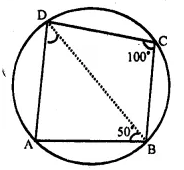=> 80° + 50° + ∠ADB = 180°
=> ∠ADB = 180° – 130° = 50°

Question 10.
Solution:
Arc BAD subtends ∠ BOD at the centre and ∠BCD at the remaining part of the circle.Question 11.
Solution:
In ∆ OAB,
OA = OB (radii of the same circle)
∠OAB = ∠OBA = 50°
and Ext ∠BOD = ∠OAB + ∠OBA
=>x° = 50° + 50° – 100°
(opposite angles of a cyclic quad.)
=> 50°+ y° = 180°
=> y° = 180° – 50° = 130°
Hence x = 100° and y = 130° Ans.

Question 12.
Solution:
Sides AD and AB of cyclic quadrilateral ABCD are produced to E and F respectively.
∠CBF = 130°, ∠CDE = x.
∠CBF + ∠CBA = 180° (Linear pair)
=> 130°+ ∠CBA = 180°
=> ∠CBA = 180° – 130° = 50°
But Ext. ∠ CDE = Interior opp. ∠ CBA (In cyclic quad. ABCD)
=> x = 50° Ans.

Question 13.
Solution:
In a circle with centre O AB is its diameter and DO || CB is drawn. ∠BCD = 120°
To Find : (i) ∠BAD (ii) ABD
(v) Show that ∆ AOD is an equilateral triangle.
(i) ABCD is a cyclic quadrilateral.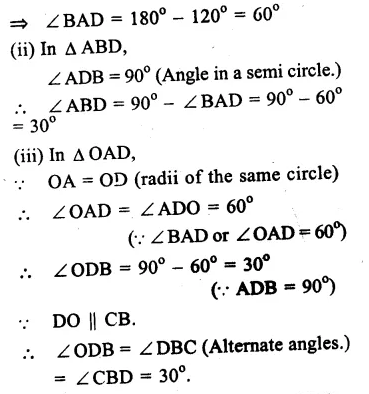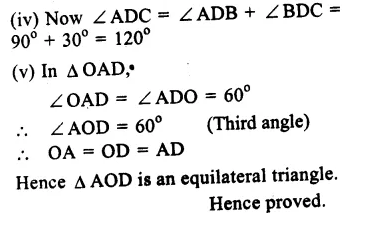Question 14.
Solution:
AB = 6cm, BP = 2cm, DP = 2.5cm
Let CD = xcm
Two chords AB and CD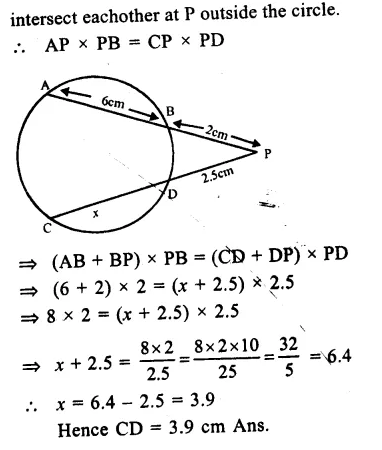Question 15.
Solution:
O is the centre of the circle
∠ AOD = 140° and ∠CAB = 50°
BD is joined.
(i) ABDC is a cyclic quadrilateral.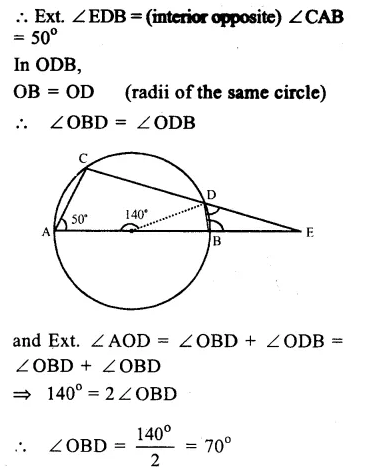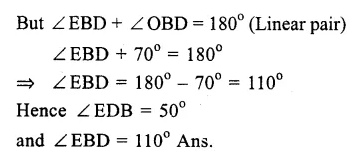Question 16.
Solution:
Given : ABCD is a cyclic quadrilateral whose sides AB and DC are produced to meet each other at E.
To Prove : ∆ EBC ~ ∆ EDA
Proof : In ∆ EBC and ∆ EDA
∠ E = ∠ E (common)
{Exterior angle of a cyclic quad, is equal to its interior opposite angle}
and ∠ EBC = ∠EDA
∆ EBC ~ ∆ EDA (AAS axiom)
Hence proved

Question 17.
Solution:
Solution Given : In an isosceles ∆ ABC, AB = AC
A circle is drawn x in such a way that it passes through B and C and intersects AB and AC at D and E respectively.
DE is joined.
To Prove : DE || BC
Proof : In ∆ ABC,
AB = AC (given)
∠ B = ∠ C (angles opposite to equal sides)
But ∠ ADE = ∠ C (Ext. angle of a cyclic quad, is equal E to its interior opposite angle)
But, these are corresponding angles
DE || BC.
Hence proved.

Question 18.
Solution:
Given : ∆ ABC is an isosceles triangle in which AB = AC.
D and E are midpoints of AB and AC respectively.
DE is joined.
To Prove : D, B, C, E are concyclic.
Proof: D and E are midpoints of sides AB and AC respectively.
DE || BC
In ∆ ABC, AB = AC
∠B = ∠C
But ∠ ADE = ∠ B (alternate angles)
But ∠ADE is exterior angle of quad. DBCE which is equal to its interior opposite angle C.
Hence D, B, C, E are con cyclic.
Hence proved.

Question 19.
Solution:
Given : ABCD is a cyclic quadrilateral whose perpendicular bisectors l, m, n, p of the side are drawnTo prove : l, m, n and p are concurrent.
Proof : The sides AB, BC, CD and DA are the chords of the circle passing through the vertices’s of quad. A, B, C and D. and perpendicular bisectors of a chord always passes through the centre of the circle.
l,m, n and p which are the perpendicular bisectors of the sides of cyclic quadrilateral will pass through O, the same point Hence, l, m, n and p are concurrent.
Hence proved.

Question 20.
Solution:
Given : ABCD is a rhombus and four circles are drawn on the sides AB, BC, CD and DA as diameters. Diagonal AC and BD intersect each other at O.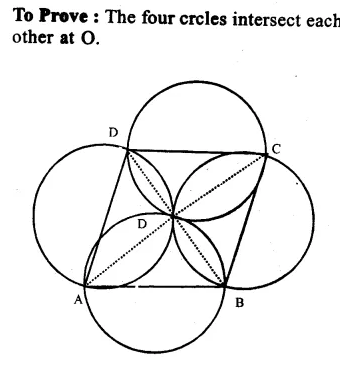Question 21.
Solution:
Given: ABCD is a rectangle whose diagonals AC and BD intersect each other at O.
To prove : O is the centre of the circle passing through A, B, C and D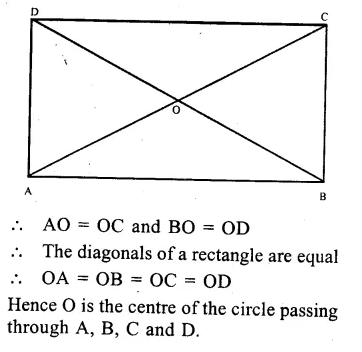Question 22.
Solution:
Construction.
(i) Let A, B and C are three points
(ii) With A as centre and BC as radius draw an arc
(iii) With centre C, and radius AB, draw another arc which intersects the first arc at D.
D is the required point.
Join BD and CD, AC and BA and CB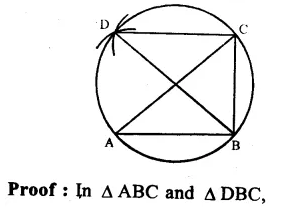BC = BC (common)
AC = BD (const.)
AB = DC
∴ ∆ ABC ≅ ∆ DBC (SSS axiom)
∴ ∠BAC ≅ ∠BDC (c.p.c.t.)
But these are angles on the same sides of BC
Hence these are angles in the same segment of a circle
A, B, C, D are concyclic Hence D lies on the circle passing througtvA, B and C.
Hence proved.

Question 23.
Solution:
Given : ABCD is a cylic quadrilateral (∠B – ∠D) = 60°
To prove : The small angle of the quad, is 60°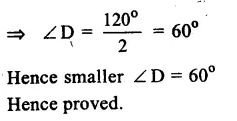Question 24.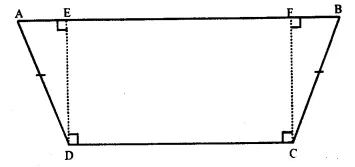Solution:
To prove : A, B, C and D lie on a circle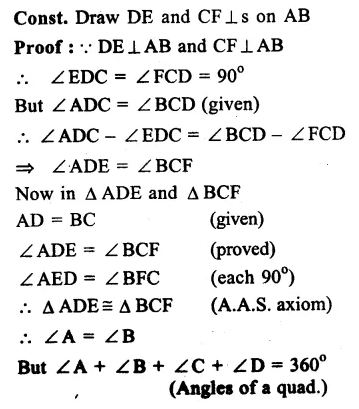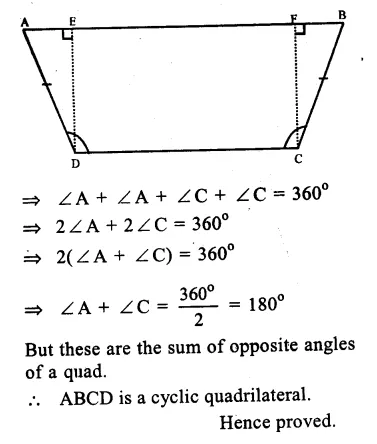Question 25.
Solution:
Given : In the figure, two circles intersect each other at D and C
∠BAD = 75°, ∠DCF = x° and ∠DEF = y°Question 26.
Solution:
Given : ABCD is a cyclic quadrilateral whose diagonals AC and BD intersect at O at right angle.Question 27.
Solution:
In a circle, two chords AB and CD intersect each other at E when produced.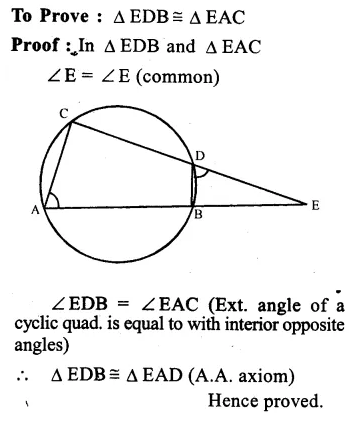Question 28.
Solution:
Given : Two parallel chords AB and CD of a circle BD and AC are joined and produced to meet at E.Question 29.
Solution:
Given : In a circle with centre O, AB is its diameter. ADE and CBE are lines meeting at E such that ∠BAD = 35° and ∠BED = 25°.
To Find : (i) ∠DBC (ii) ∠DCB (iii) ∠BDC
Solution. Join BD and AC,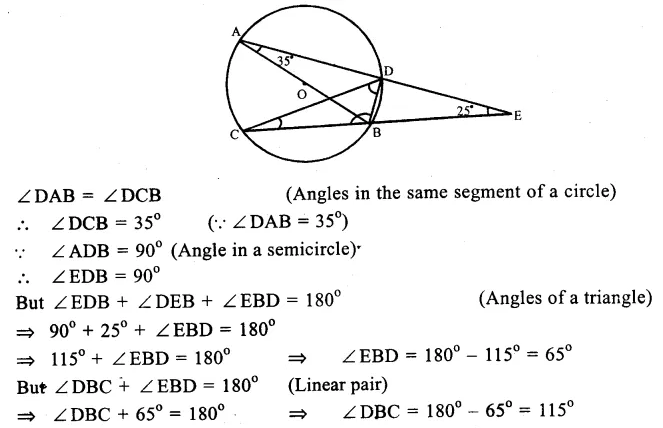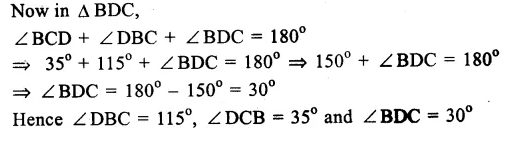Hope given RS Aggarwal Solutions Class 9 Chapter 11 Circle Ex 11C are helpful to complete your math homework.

If you have any doubts, please comment below. Learn Insta try to provide online math tutoring for you.